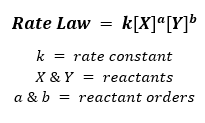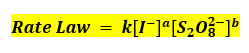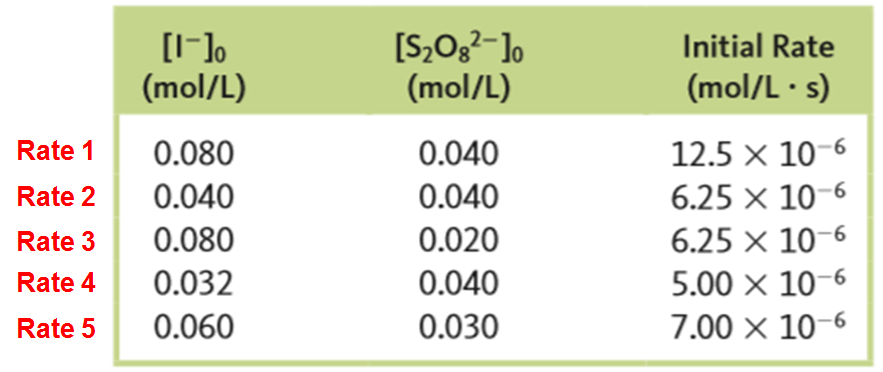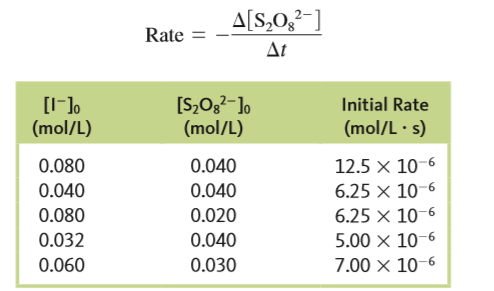# Problem: The reaction2I- (aq) + S2O82- (aq) → I2 (aq) + 2SO42- (aq)was studied at 25°C. The following results were obtained wherea. Determine the rate law.

###### FREE Expert Solution

Recall that rate law focuses only on the concentrations of the reactants.Rate law for the reaction:Calculating reactant order:

→ look for the data where the concentration of one changes while the other remains constant

Calculate order with respect to I- (a):

79% (227 ratings)###### Problem Details

The reaction

2I- (aq) + S2O82- (aq) → I(aq) + 2SO42- (aq)

was studied at 25°C. The following results were obtained wherea. Determine the rate law.